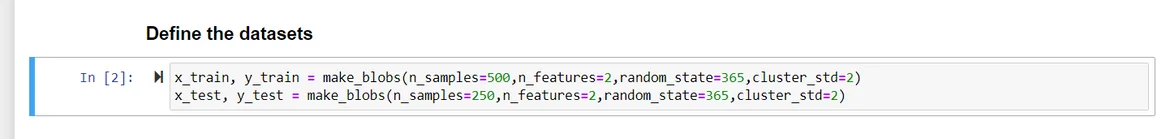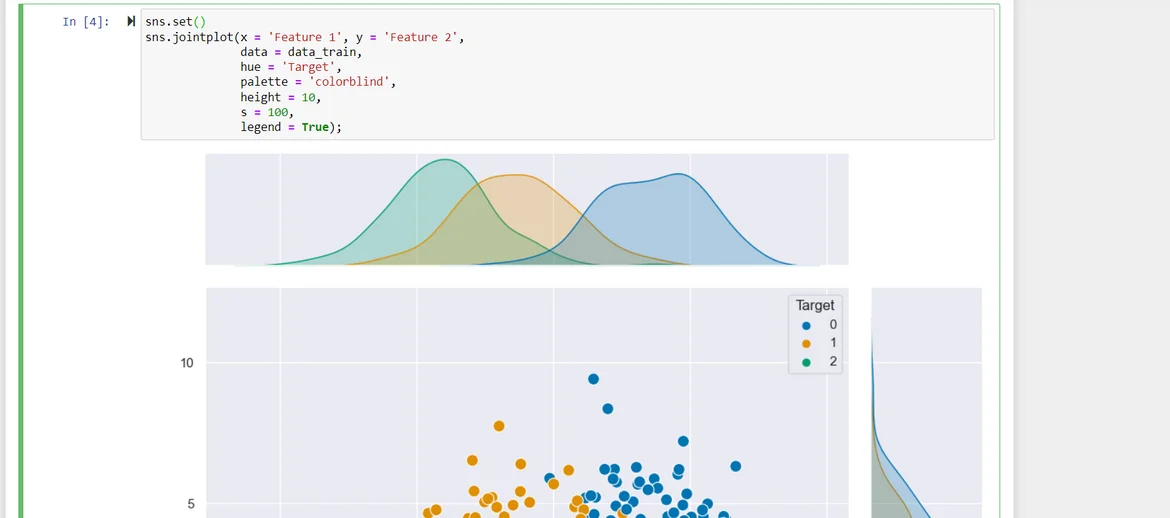13 Sept 2022

Posted on:

10 Sept 2022

0

# Resolved:Course Exam Question 1 for Machine learning with KNN

Hi,
I would like ask about the first question in the course exam
the question mentioned that the data must be defined to 5 distinct classes without giving the centers
I tried a lot but I can't solve the problem

Posted on:

12 Sept 2022

0

Hey Amro,

Thank you for reaching out!

The question asks to define the training and test sets separately. Therefore, you would need to call the `make_blobs` function twice - once for defining the training dataset and once for defining the test set.

Inside each `make_blobs` function, you would need to define the following:
1. the number of representatives from each class
2. the number of features
3. the standard deviation of the clusters
4. as well as the random state.
The random state would take care of the centers, so you don't need to specify them in this exercise.

Hope this helps!

Kind regards,
365 Hristina

Posted on:

12 Sept 2022

0

Hi Hristina,
Thank you for your quick response!

Can you please advise as I defined the same as you mentioned and number of targets is 3 instead of 5BR,
Amro

Posted on:

13 Sept 2022

0

Hey again Amro,

Notice how the n_samples parameter is implemented. The description in the documentation is as follows:
If int, it is the total number of points equally divided among clusters. If array-like, each element of the sequence indicates the number of samples per cluster.
Therefore, by passing 500 as an argument, you give the total number of points, which `make_blobs` then distributes (roughly) equally among 3 classes. Classes 0 and 1 have 167 representatives while class 2 has 166. You can convince yourself that this is the case by typing for example:

``````import numpy as np
np.count_nonzero(y_train == 1)
``````

In order to perform the task from the exam successfully, you would need to pass an array as an argument which explicitly states the number of classes and the number of representatives from each class. We need 5 classes, with 100 representatives each. Therefore, the following would work:

``````n_samples = [100, 100, 100, 100, 100]
``````

or, written in a more compact way:

``````n_samples = *5
``````

You would then need to do this analogously for the test dataset, this time having 50 representatives from each of the 5 classes. The rest of the parameters (`n_features`, `random_state`, and `cluster_std`) are defined correctly in your code.

Hope this helps!

Kind regards,
365 Hristina

Posted on:

13 Sept 2022

0

Got it